### IMO Shortlist 1996 problem C1

Kvaliteta:
Avg: 0,0
Težina:
Avg: 6,0
We are given a positive integer$r$ and a rectangular board$ABCD$ with dimensions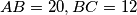$AB = 20, BC = 12$. The rectangle is divided into a grid of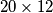$20 \times 12$ unit squares. The following moves are permitted on the board: one can move from one square to another only if the distance between the centers of the two squares is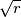$\sqrt {r}$. The task is to find a sequence of moves leading from the square with$A$ as a vertex to the square with$B$ as a vertex.

(a) Show that the task cannot be done if$r$ is divisible by 2 or 3.

(b) Prove that the task is possible when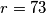$r = 73$.

(c) Can the task be done when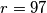$r = 97$?
Izvor: Međunarodna matematička olimpijada, shortlist 1996